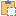#### Rotate Method

Rotate the element within the project by a specified angle around a given axis.

Namespace: Autodesk.Revit.DB
Assembly: RevitAPI (in RevitAPI.dll) Version: 2015.0.0.0 (2015.0.0.0)

# Syntax

C#
``````public bool Rotate(
Line axis,
double angle
)``````
Visual Basic
``````Public Function Rotate ( _
axis As Line, _
angle As Double _
) As Boolean``````
Visual C++
``````public:
bool Rotate(
Line^ axis,
double angle
)``````

#### Parameters

axis
Type: Autodesk.Revit.DB Line
An unbounded line that represents the axis of rotation.
angle
Type: System Double
The angle, in radians, by which the element is to be rotated around the specified axis.

#### Return Value

If the element is rotate successfully then the method returns True, otherwise False.

# Remarks

The rotate method is used to rotate an element within the project. Other elements may also be rotated when this element is rotated because they are dependent upon the element being rotated. An unbounded line for the axis can be created by using the Application.Create object and its methods.

# ExamplesCopy C#
``````bool LocationRotate(Autodesk.Revit.ApplicationServices.Application application, Autodesk.Revit.DB.Element element)
{
bool rotated = false;
// Rotate the element via its location curve.
LocationCurve curve = element.Location as LocationCurve;
if (null != curve)
{
Curve line = curve.Curve;
XYZ aa = line.GetEndPoint(0);
XYZ cc = new XYZ(aa.X, aa.Y, aa.Z + 10);
Line axis = Line.CreateBound(aa, cc);
rotated = curve.Rotate(axis, Math.PI / 2.0);
}

return rotated;
}``````Copy VB.NET
``````Private Function LocationRotate(application As Autodesk.Revit.ApplicationServices.Application, element As Autodesk.Revit.DB.Element) As Boolean
Dim rotated As Boolean = False
' Rotate the element via its location curve.
Dim curve As LocationCurve = TryCast(element.Location, LocationCurve)
If curve IsNot Nothing Then
Dim line__1 As Curve = curve.Curve
Dim aa As XYZ = line__1.GetEndPoint(0)
Dim cc As New XYZ(aa.X, aa.Y, aa.Z + 10)
Dim axis As Line = Line.CreateBound(aa, cc)
rotated = curve.Rotate(axis, Math.PI / 2.0)
End If

Return rotated
End Function``````